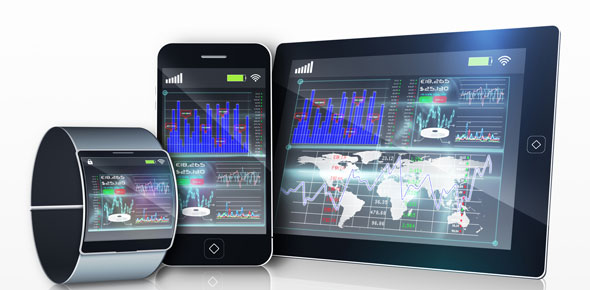# Digital Media Quiz

15 Questions | Attempts: 1736
ShareSettingsAnswer the following questions after reading the DIGITAL MEDIA pages posted on the class web site.

• 1.
A *halftone* is a pattern of tiny dots used by
• A.

Newspapers

• B.

Books

• C.

Magazines

• D.

All of the above

• E.

None of the above

• 2.
Computers reproduce photos by breaking the image up into small squares called
• A.

Pixels

• B.

Bytes

• C.

Bits

• 3.
Black and white pictures require less information because all that needs to be recorded is its position and its
• A.

Color information

• B.

• C.

Location

• 4.
A color pixel needs three numbers to define its color: one number each to indicate how much
• A.

Cyan, magenta, yellow

• B.

Red, yellow, blue

• C.

Red, green, and blue

• 5.
Black and white images require more computer file space than color images.
• A.

True

• B.

False

• 6.
A RASTER image is a rectangular grid of
• A.

Lines

• B.

Dots

• C.

Pixels

• D.

All of the above

• 7.
VECTOR graphics are based on
• A.

Color depth

• B.

Mathematical equations

• C.

Photographs

• 8.
Adobe Illustrator is a software program that uses
• A.

Mathematical equations

• B.

Vector graphics

• C.

Bezier curves

• D.

Anchor points

• E.

None of the above

• F.

All of the above

• 9.
The total number of pixels in an image is referred to as the image's
• A.

Resolution

• B.

Raster

• C.

Color depth

• D.

Both A and C

• E.

None of the above

• 10.
A JPEG image is a "lossy" format, which means that
• A.

The number of pixels in the image can be increased without loss of quality.

• B.

The image is compressed each time it is saved and could degrade.

• 11.
A TIFF image is most often used for work that is printed and saves
• A.

8 bits per color

• B.

16 bits per color

• C.

Both A and B

• 12.
Images in RAW format is "lossless"
• A.

True

• B.

False

• 13.
A GIF image uses a limited color palette and is best used for
• A.

Simple shapes and cartoon-like images

• B.

Printing photographs

• C.

Halftones

• 14.
PNG images are "lossless" and therefore their file size is ______________ than JPEG images.
• A.

Smaller

• B.

Larger

• 15.
BMP files are large files developed for use mostly with
• A.

Windows programs

• B.

Macintosh programs

• C.

Scanners

• D.

Both B and C

• E.

Both A and C

## Related TopicsBack to top
×

Wait!
Here's an interesting quiz for you.